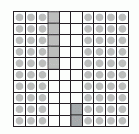Email us to get an instant 20% discount on highly effective K-12 Math & English kwizNET Programs!

Online Quiz (WorksheetABCD)

Questions Per Quiz = 2 4 6 8 10

Grade 5 - Mathematics Decimal Sums (WIZ Math)

 Write the decimals one below the other. Note: The point must be aligned and the numbers one below the other. Example: Add 0.35 and 0.42 Consider a square being divided into 100 equal parts.Shade 3 tenths and colour five hundreths which shows 0.35. Shade 4 tenths and colour 2 hundreths which shows 0.42. Count the total number of tenths and hundreths in the square.Answer: 0.77 Directions: Add the following decimal numbers. Also write at least ten examples of your own.
 Question 1: 6.8091 + 1.4591

 Question 2: 19.751 + 86.183

 Question 3: 519.23 + 59.81

 Question 4: 6589.0 + 8565.4

 Question 5: 414.0 + 804.8

Question 6: This question is available to subscribers only!

Question 7: This question is available to subscribers only!

Question 8: This question is available to subscribers only!

Question 9: This question is available to subscribers only!

Question 10: This question is available to subscribers only!